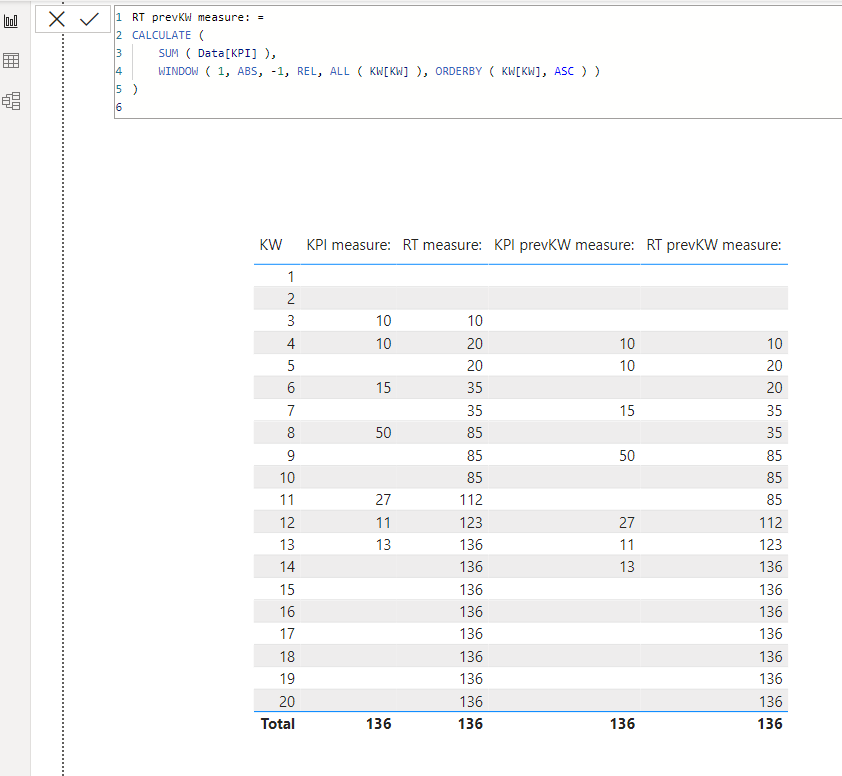cancel
Showing results for
Did you mean:New Member

## Running total by previous week

Hello,

I tried to solve a running total problem that is driving me mad. A running total is fine; it can even be solved with a quick measure, but this is a little trickier.

The needed measure should show the running total by previous week. E.G. the calender week 14 shows the runnig total for KW 13. The screenshot below shows that there is a KPI by calendar week (column 1) & and a working running total for it (column 2). The third colums shifts the KPI value by 1 week.I copied the working formula out of column 2 and changed the included measure, but it does not work. There is no result (column 4). This is what the database looks like:This is the non-working running total (column 4). It works when I use KPI 1 (column1)

``````KPI +1 KW RT =
CALCULATE(
[KPI + 1 KW],
FILTER(
ALLSELECTED('tbl_datetable'[date]),
ISONORAFTER('tbl_datetable'[date], MAX('tbl_datetable'[date]), DESC)
)
)``````

Help would be much apreciated.
Eric

1 ACCEPTED SOLUTIONSuper User

Hi,

I tried to create a sample pbix file like below.

Please check the below picture and the attached pbix file.``````KPI measure: =
SUM( Data[KPI] )``````
``````RT measure: =
CALCULATE (
SUM ( Data[KPI] ),
WINDOW ( 1, ABS, 0, REL, ALL ( KW[KW] ), ORDERBY ( KW[KW], ASC ) )
)
``````
``````KPI prevKW measure: =
CALCULATE (
SUM ( Data[KPI] ),
OFFSET ( -1, ALL ( KW[KW] ), ORDERBY ( KW[KW], ASC ) )
)
``````
``````RT prevKW measure: =
CALCULATE (
SUM ( Data[KPI] ),
WINDOW ( 1, ABS, -1, REL, ALL ( KW[KW] ), ORDERBY ( KW[KW], ASC ) )
)
``````

If this post helps, then please consider accepting it as the solution to help other members find it faster, and give a big thumbs up.

2 REPLIES 2New Member

Hi Jihwan_Kim,
it works. Many thanks.
The window function is something I haven't encountered before. Tomorrow, I will examine your solution more closely and experiment with it.

EricSuper User

Hi,

I tried to create a sample pbix file like below.

Please check the below picture and the attached pbix file.``````KPI measure: =
SUM( Data[KPI] )``````
``````RT measure: =
CALCULATE (
SUM ( Data[KPI] ),
WINDOW ( 1, ABS, 0, REL, ALL ( KW[KW] ), ORDERBY ( KW[KW], ASC ) )
)
``````
``````KPI prevKW measure: =
CALCULATE (
SUM ( Data[KPI] ),
OFFSET ( -1, ALL ( KW[KW] ), ORDERBY ( KW[KW], ASC ) )
)
``````
``````RT prevKW measure: =
CALCULATE (
SUM ( Data[KPI] ),
WINDOW ( 1, ABS, -1, REL, ALL ( KW[KW] ), ORDERBY ( KW[KW], ASC ) )
)
``````

If this post helps, then please consider accepting it as the solution to help other members find it faster, and give a big thumbs up.